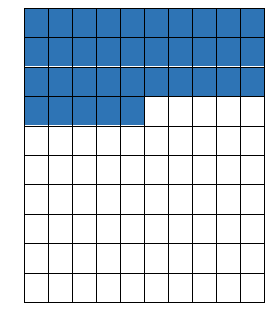# Math Worksheets Land

Math Worksheets For All Ages

# Math Worksheets Land

Math Worksheets For All Ages

Home > Topics >

# Percentage Worksheets

What are Percentages? You can observe percentages almost everywhere, in shops and supermarkets, advertising and even on the internet. Percentages are one of the essential concepts that form the basis of many advanced mathematical concepts. Students must have a firm grip on this concept to help them gain a better understanding of future concepts. The basic learning of percentages begins in third grade which then steadily advances in future grades. To fully grasp the concept, students should be able to understand the relationship of percentage with decimals and fractions. The term 'percent' stands for 'out of hundred'. Percentages, like decimals and fractions, are used to describe the parts of the whole. In percentage, the whole is divided into a hundred equal parts. Percentages are denoted by the symbol '%'. To help your students learn percentages easily, try using 100 small boxes. You can use the grid to represent the whole and parts of percentages. If you color the 25 boxes out of the 100 boxes, then students can visualize 15%. If you color all the 100 boxes, you can demonstrate 100% to the students. Encourage students to design their grids and compute different percentages by coloring different numbers of boxes. You will work through all kinds of conversions with percentages. I like to call them the "Math Conversion Trilogy": Decimals, Fractions, and Percentage. You will move between all three very often.

### Best Practices for Teaching Students the Concept of Percentage

The goal here is to help students understand how much of each unit in a group contributes towards a full unit. This is a fundamental concept that all students will need to understand to become productive citizens. Here is the progression of lessons we have found to really help students solidify their understanding of this concept:One Hundred Grids - Start with a 10 x 10 (100) grid to pass this concept on to students. The 100 grid is the whole and the part represents the amount of the part that you have in hand. A good starting point is a value that is less than half, such as 35%. That value would fill the first 3 rows and half of the 4th row so as the image to the right portrays.

From here you could move on to a value that is greater than a half. This art-based approach really helps students visualize the concept of a whole and the parts that have been occupied within the whole based on the percentage that is given. We encourage you to continue on with this form until it becomes second nature for them.Percentage of a Value - Then we move the visual to a tag diagram to best understand the progress towards a whole unit. This leads us to using a rectangular shape which we estimate a portion of the whole. If we were to find the value 35% towards a whole, it would look like what you see to the right.

This then progresses us towards the use of math. We introduce students the concept that a percentage is a decimal value based on the logic that 1 is equal to 100%. That means that 35% is equal to 0.35, when observed from a 100% view. This means that if we were asked what is 35% of 60, it would be the same as 0.35 x 60 or 21. This progression carries on to any value. Take the percentage and just divide by 100.

Unlock all the answers, worksheets, homework, tests and more!
Save Tons of Time! Make My Life Easier Now

## Thanks and Don't Forget To Tell Your Friends!

I would appreciate everyone letting me know if you find any errors. I'm getting a little older these days and my eyes are going. Please contact me, to let me know. I'll fix it ASAP.